Leverage Point and Outlier Detection

The regular variable LEVERAGE is defined as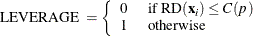where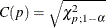is the cutoff value.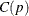can be set with the leverage CUTOFF= option, andcan be set with the leverage CUTOFFALPHA= option.

If projected robust distances are computed for a data set that has a low-dimensional structure, the default cutoff value is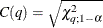where q is the dimensionality of the low-dimensional space. The LEVERAGE is then defined aswhere POD is the projected off-plane distance and PRD denotes the projected robust distance. You can specify a cutoff value with the CUTOFF or the CUTOFFALPHA suboptions of the LEVERAGE option in the MODEL statement.

Residuals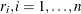, based on robust regression estimates are used to detect vertical outliers. The variable OUTLIER is defined as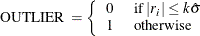where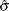is the estimated scale in the model and the multiplierof the cutoff value is specified by the CUTOFF= option in the MODEL statement. By default, k = 3.

An ODS table called Diagnostics contains the LEVERAGE and OUTLIER variables.